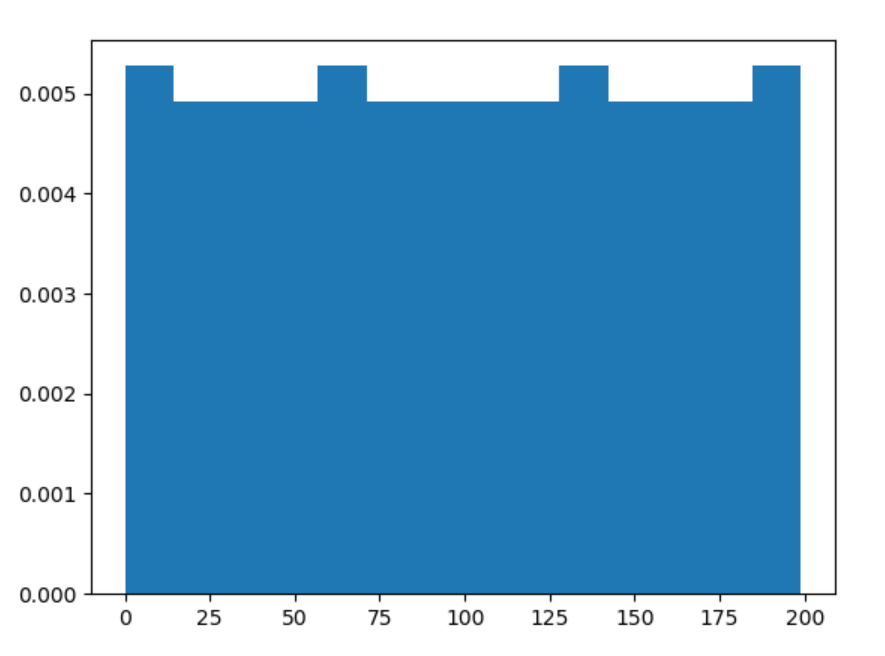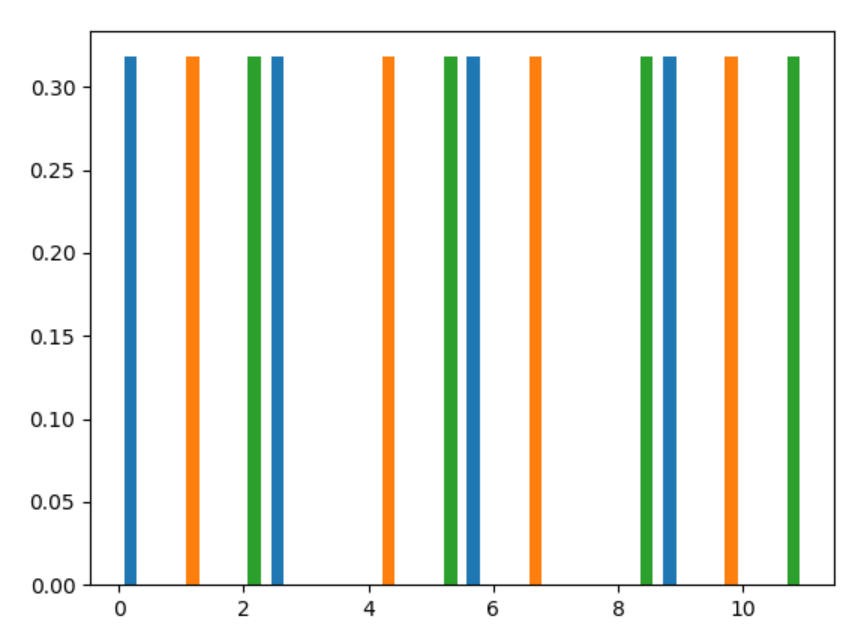Related Articles

# numpy.random.permutation() in Python

• Last Updated : 15 Jul, 2020

With the help of numpy.random.permutation() method, we can get the random samples of sequence of permutation and return sequence by using this method.

Syntax : numpy.random.permutation(x)

Return : Return the random sequence of permuted values.

Example #1 :

In this example we can see that by using numpy.random.permutation() method, we are able to get the sequence of permutation and it will return the sequence by using this method.

## Python3

 `# import numpy``import` `numpy as np``import` `matplotlib.pyplot as plt`` ` `# Using permutation() method``gfg ``=` `np.random.permutation(``200``)`` ` `count, bins, ignored ``=` `plt.hist(gfg, ``14``, density ``=` `True``)``plt.show()`

Output :Example #2 :

## Python3

 `# import numpy``import` `numpy as np``import` `matplotlib.pyplot as plt`` ` `arr ``=` `np.arange(``12``).reshape((``4``, ``3``))``# Using permutation() method``gfg ``=` `np.random.permutation(arr)`` ` `count, bins, ignored ``=` `plt.hist(gfg, ``14``, density ``=` `True``)``plt.show()`

Output :Attention geek! Strengthen your foundations with the Python Programming Foundation Course and learn the basics.

To begin with, your interview preparations Enhance your Data Structures concepts with the Python DS Course. And to begin with your Machine Learning Journey, join the Machine Learning – Basic Level Course

My Personal Notes arrow_drop_up